The availability of S Chand Class 9 Maths Solutions ICSE Chapter 16 Area of Plane Figures Chapter Test encourages students to tackle difficult exercises.

## S Chand Class 9 ICSE Maths Solutions Chapter 16 Area of Plane Figures Chapter Test

Question 1.
What is the area of an equilateral triangle having altitude equal to 2√3 cm?
(a) √3cm2
(b) 2√3cm2
(c) 3√3cm2
(d) 4√3cm2
Solution:
Altitude = $$\frac{\sqrt{3}}{2}$$ sides ⇒ $$\frac{\sqrt{3}}{2}$$ = 2√3 cm
∴ Side = $$\frac{2 \sqrt{3} \times 2}{\sqrt{3}}$$ = 4 cm
Area = $$\frac{\sqrt{3}}{4}$$ side2 = $$\frac{\sqrt{3}}{4}$$ × 4 × 4
= 4√3cm2 (d)

Question 2.
The altitude of an equilateral triangle is √3 cm. What is its perimeter?
(a) 3 cm
(b) 3√3 cm
(c) 6 cm
(d) 6√3 cm
Solution:
Altitude of an equilateral triangle = √3
∴ $$\frac{\sqrt{3}}{2}$$ side = √3 ⇒ side = 2 cm
and perimeter = 3 × Side
=3 × 2 = 6 cm

Question 3.
The sides of a triangle are in the ratio 2 : 3 : 4. The perimeter of the triangle is 18 cm. The area (in cm2) of the triangle is
(a) 9
(b) 36
(c) √42
(d) 6√15
Solution:
Sides of a triangle are in the ratio = 2 : 3 : 4
and perieter = 18 cm
Sum of ratios = 2 + 3 + 4 = 9
∴ First side = $$\frac { 18 }{ 9 }$$ × 2 = 4 cm
Second side = $$\frac { 18 }{ 9 }$$ × 3 = 6 cm
Third side = $$\frac { 18 }{ 9 }$$ × 4 = 8 cm
∴ s = $$\frac{a+b+c}{2}$$
= $$\frac { 18 }{ 9 }$$ = 9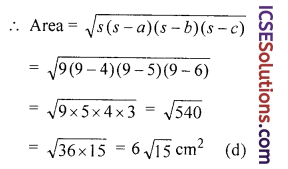Question 4.
A parallelogram has sides 15 cm and 7 cm long. The length of one of the diagonals is 20 cm. The area of the parallelogram is
(a) 42 cm2
(b) 60 cm2
(c) 84 cm2
(d) 96 cm2
Solution:
Sides of a parallelogram = 15 cm and 7 cm
and length of one diagonal = 20 cm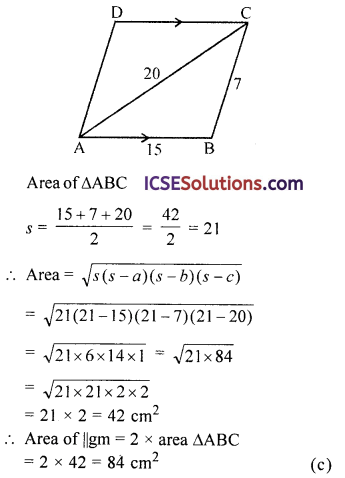Question 5.
The perimeter of two squares are 68 cm and 60 cm. The perimeter of a third square whose area is equal to the difference of the areas of the two squares.
(a) 64 cm
(b) 60 cm
(c) 32 cm
(d) 8 cm
Solution:
Perimeter of one square = 68 cm
∴ Side = $$\frac { 68 }{ 4 }$$ = 17 cm
Perimeter of second square = 60 cm
∴ Side = $$\frac { 60 }{ 4 }$$ = 15 cm
Area of first square = (Side)2
= (17)2 = 289 cm2
Area of first square = (Side)2
=(17)2 = 289 cm2

Area second square = (15) = 225 cm2
∴ Area of third square = 289 – 225 = 64 cm2
∴ Side = $$\sqrt{\text { Area }}$$ = $$\sqrt{64}$$ = 8 cm
and perimeter =4 × side = 4 × 8 = 32 cm (c)

Question 6.
A square is of area 200 m2. A new square is formed in such a way that the length of its diagonal is √2 times of the diagonal of the given square. Then the area of the new square formed is
(a) 200√2 sq m
(b) 400√2 sq m
(c) 400 sq m
(d) 800 sq m
Solution:
Area of a square = 200 m2
∴ Its diagonal = $$\sqrt{\text { Area } \times 2}$$
= $$\sqrt{200 \times 2}$$ = $$\sqrt{400}$$ = 20 m
∴ Diagonal of second square = √2 × 20 m
Area of second square = $$\frac{(\text { diagonal })^2}{2}$$
= $$\frac{(\sqrt{2} \times 20)^2}{2}$$ = $$\frac{2 \times 400}{2}$$ = 400 m2 (c)

Question 7.
The sides of a parallelogram are in the ratio 5 : 4. Its area is 1000 sq. units. Altitude on the greater side is 20 units. Altitude on the smaller side is
(a) 30 units
(b) 25 units
(c) 10 units
(d) 15 units
Solution:
Ratio in the sides of a parallelogram = 5 : 4
Area = 1000 sq. units
Altitude on the greater side = 20 units
Let greater side = 5x
and smaller side = 4x
∴ Area = Greater side × Altitude on it
1000 = 5x × 20 ⇒ x = $$\frac{1000}{5 \times 20}$$ = 10
∴ Greater side = 5 × 10 = 50 units
and altitude on smaller side = $$\frac{\text { Area }}{\text { Smaller side }}$$ = $$\frac{1000}{4 \times 10}$$ = 25 units

Question 8.
One diagonal and perimeter of a rhombus are 24 cm and 52 cm respectively. The other diagonal is
(a) 13 cm
(b) 12 cm
(c) 10 cm
(d) 15 cm
Solution:
Perimeter of a rhombus = 52 cm
∴ Side = $$\frac{\text { Perimeter }}{4}$$ = $$\frac{52}{4}$$ = 13 cm
One diagonal = 24 cm
By joining the diagonals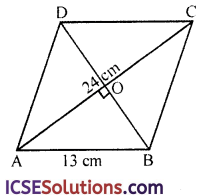They bisect each other at O at right angle
∴ AO = OC = $$\frac { 24 }{ 2 }$$ = 12 cm and BO = OD
In right △AOB
AB2 = AO2 + BO2
⇒ (13)2 = 122 + B2
⇒ 169 = 144 + B2
⇒ BO2 = 169 – 144 = 25 = (5)2
BO = 5 cm
∴ Diagonal BD = 2 × BO
= 2 × 5 = 10 cmQuestion 9.
The area of a field in the shape of a trapezium measures 1440 m2. The perpendicular distance between its parallel sides is 24 m. If the ratio of the parallel sides is 5 : 3, the length of the longer parallel sides is:
(a) 45 m
(b) 60 m
(c) 75 cm
(d) 120 m
Solution:
Area of a trapezium shaped field = 1440 m2
Perpendicular distance = 24 m
∴ Sum of parallel sides = $$\frac{\text { Area } \times 2}{\text { Height }}$$ = $$\frac{1440 \times 2}{24}$$ = 120 cm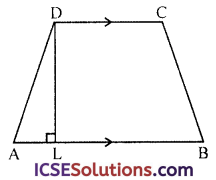Ratio in side = 5 : 3
Sum of ratio = 5 + 3 = 8
∴ First longer side = $$\frac { 120 }{ 8 }$$ × 5 = 75 m

Question 10.
A lawn 30 m long and 16 m wide is surrounded by a path 2 m wide. What is the area of the path?
(a) 200 m2
(b) 280 m2
(c) 300 m2
(d) 320 m2
Solution:
Length of a lawn = 30 m
Breadth of a lawn = 16 m
Width of path around it = 2 m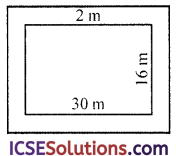∴ Outer length = 30 + 2 × 2
= 30 + 4 = 34 m
and outer breadth = 16 + 2 × 2
=16 + 4 = 20 m
∴ Area of path = Outer Area – Inner area
= 34 × 20 – 30 × 16 m2
& = 680 – 480 = 200 m2 (a)